Next: Worked example 4.4: Suspended Up: Newton's laws of motion Previous: Worked example 4.2: Block

## Worked example 4.3: Raising a platform

Question: Consider the diagram. The platform and the attached frictionless pulley weigh a total of 34N. With what forcemust the (light) rope be pulled in order to lift the platform at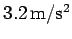?Answer: Let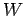be the weight of the platform,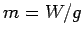the mass of the platform, and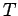the tension in the rope. From Newton's third law, it is clear that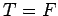. Let us apply Newton's second law to the upward motion of the platform. The platform is subject to two vertical forces: a downward forcedue to its weight, and an upward force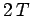due to the tension in the rope (the force is, rather than, because both the leftmost and rightmost sections of the rope, emerging from the pulley, are in tension and exerting an upward force on the pulley). Thus, the upward accelerationof the platform is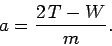Sinceand, we obtain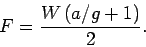Finally, given that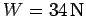and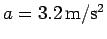, we have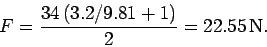Next: Worked example 4.4: Suspended Up: Newton's laws of motion Previous: Worked example 4.2: Block
Richard Fitzpatrick 2006-02-02